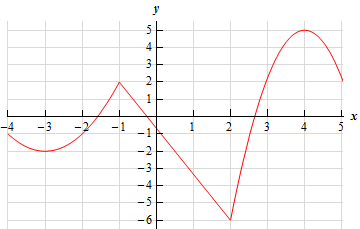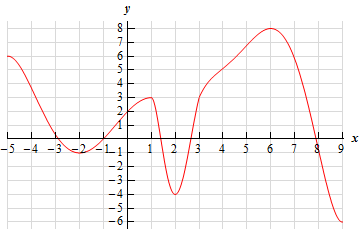Paul's Online Notes
Home / Calculus I / Applications of Derivatives / Minimum and Maximum Values
Show Mobile Notice Show All Notes Hide All Notes
Mobile Notice
You appear to be on a device with a "narrow" screen width (i.e. you are probably on a mobile phone). Due to the nature of the mathematics on this site it is best views in landscape mode. If your device is not in landscape mode many of the equations will run off the side of your device (should be able to scroll to see them) and some of the menu items will be cut off due to the narrow screen width.

### Section 4-3 : Minimum and Maximum Values

1. Below is the graph of some function, $$f\left( x \right)$$. Identify all of the relative extrema and absolute extrema of the function.Solution
2. Below is the graph of some function, $$f\left( x \right)$$. Identify all of the relative extrema and absolute extrema of the function.Solution
3. Sketch the graph of $$g\left( x \right) = {x^2} - 4x$$ and identify all the relative extrema and absolute extrema of the function on each of the following intervals.
1. $$\left( { - \infty ,\infty } \right)$$
2. $$\left[ { - 1,4} \right]$$
3. $$\left[ {1,3} \right]$$
4. $$\left[ {3,5} \right]$$
5. $$\left( { - 1,5} \right]$$
Solution
4. Sketch the graph of $$h\left( x \right) = - {\left( {x + 4} \right)^3}$$and identify all the relative extrema and absolute extrema of the function on each of the following intervals.
1. $$\left( { - \infty ,\infty } \right)$$
2. $$\left[ { - 5.5, - 2} \right]$$
3. $$\left[ { - 4, - 3} \right)$$
4. $$\left[ { - 4, - 3} \right]$$
Solution
5. Sketch the graph of some function on the interval $$\left[ {1,6} \right]$$ that has an absolute maximum at $$x = 6$$ and an absolute minimum at $$x = 3$$. Solution
6. Sketch the graph of some function on the interval $$\left[ { - 4,3} \right]$$ that has an absolute maximum at $$x = - 3$$ and an absolute minimum at $$x = 2$$. Solution
7. Sketch the graph of some function that meets the following conditions :
1. The function is continuous.
2. Has two relative minimums.
3. One of relative minimums is also an absolute minimum and the other relative minimum is not an absolute minimum.
4. Has one relative maximum.
5. Has no absolute maximum.
Solution